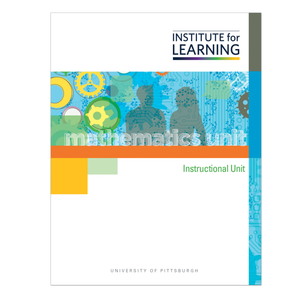# High School: Data Analysis (CCSSM Version)

Regular price \$149.00 \$0.00 Unit price per

Concepts

• Understand and Use a Normal Distribution
• Collect and Use Data to Make Inferences About a Population
• Simulation and Inference with Sample Proportions

Unit includes

• 15 high-level tasks with associated QuickStarts, Practices, Applications, and Quick Writes.
• 3 checkpoints and 6 support activities.
• 1 final performance-based assessment with scoring guide.

Students

• Determine probability using geometric areas, ratios, and proportions.
• Apply the concept of area to a normal distribution curve to find the probability of an interval under the normal curve by making a ratio of the area of the interval to the area under the entire curve.
• Use the empirical rule to estimate the probability of events from a normal distribution.
• Determine probability using geometric areas, ratios, and proportions.
• Apply the concept of area to a normal distribution curve to find the probability of an interval under the normal curve by making a ratio of the area of the interval to the area under the entire curve.
• Use the empirical rule to estimate the probability of events from a normal distribution.
• Determine probability using geometric areas, ratios, and proportions.
• Apply the concept of area to a normal distribution curve to find the probability of an interval under the normal curve by making a ratio of the area of the interval to the area under the entire curve.
• Use the empirical rule to estimate the probability of events from a normal distribution.